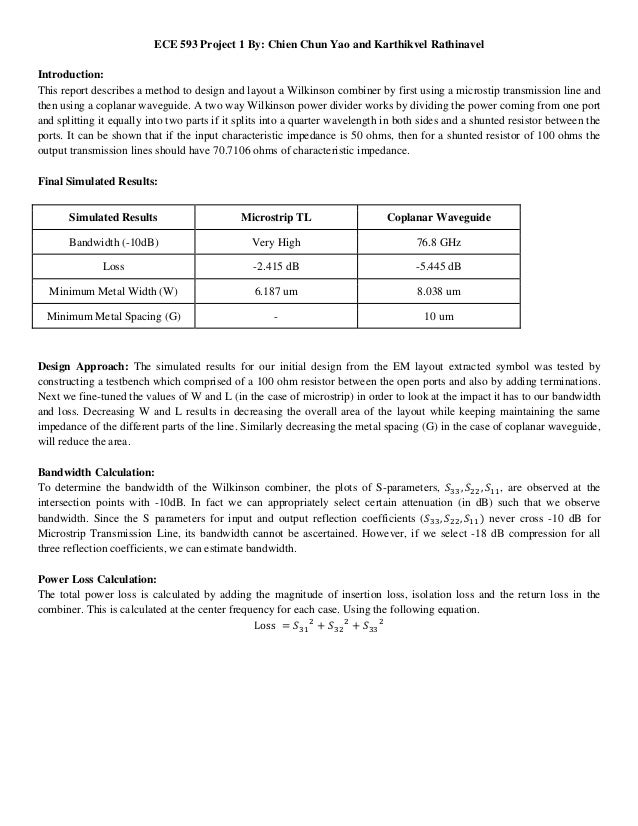Successfully reported this slideshow.
We use your LinkedIn profile and activity data to personalize ads and to show you more relevant ads. You can change your ad preferences anytime.Upcoming SlideShare
Loading in …5
×

# Ece593 project1 chien_chun_yao_and_karthikvel_rathinavel

• Designed a Wilkinson Combiner at 30 GHz using microstrip transmission line and then at 60 GHz using coplanar waveguide.
• Simulated the Layout of the testbench using the EM Simulator at RF.

• Full Name
Comment goes here.

Are you sure you want to Yes No
Your message goes here• Login to see the comments

• Be the first to like this

### Ece593 project1 chien_chun_yao_and_karthikvel_rathinavel

1. 1. ECE 593 Project 1 By: Chien Chun Yao and Karthikvel Rathinavel Introduction: This report describes a method to design and layout a Wilkinson combiner by first using a microstip transmission line and then using a coplanar waveguide. A two way Wilkinson power divider works by dividing the power coming from one port and splitting it equally into two parts if it splits into a quarter wavelength in both sides and a shunted resistor between the ports. It can be shown that if the input characteristic impedance is 50 ohms, then for a shunted resistor of 100 ohms the output transmission lines should have 70.7106 ohms of characteristic impedance. Final Simulated Results: Simulated Results Microstrip TL Coplanar Waveguide Bandwidth (-10dB) Very High 76.8 GHz Loss -2.415 dB -5.445 dB Minimum Metal Width (W) 6.187 um 8.038 um Minimum Metal Spacing (G) - 10 um Design Approach: The simulated results for our initial design from the EM layout extracted symbol was tested by constructing a testbench which comprised of a 100 ohm resistor between the open ports and also by adding terminations. Next we fine-tuned the values of W and L (in the case of microstrip) in order to look at the impact it has to our bandwidth and loss. Decreasing W and L results in decreasing the overall area of the layout while keeping maintaining the same impedance of the different parts of the line. Similarly decreasing the metal spacing (G) in the case of coplanar waveguide, will reduce the area. Bandwidth Calculation: To determine the bandwidth of the Wilkinson combiner, the plots of S-parameters, , , , are observed at the intersection points with -10dB. In fact we can appropriately select certain attenuation (in dB) such that we observe bandwidth. Since the S parameters for input and output reflection coefficients ( , , never cross -10 dB for Microstrip Transmission Line, its bandwidth cannot be ascertained. However, if we select -18 dB compression for all three reflection coefficients, we can estimate bandwidth. Power Loss Calculation: The total power loss is calculated by adding the magnitude of insertion loss, isolation loss and the return loss in the combiner. This is calculated at the center frequency for each case. Using the following equation. Loss
2. 2. Testbench for extracted EM simulation:
3. 3. A. A single-ended 30GHz Wilkinson Combiner using a mircrostrip transmission line. Schematic: Layout:
4. 4. Simulations for loss and bandwidth from extracted EM simulation for Microstrip Transmission Line: Discussion To increase the bandwidth, the number of input ports could be increased; stepped multiple sections (Pozar). Increasing numbers of input ports leads to high power loss, which implies larger bandwidth. Area could be further decreased by decreasing the length of each MLIN. This could be done by decreasing the angle while for the same line impedance by performing lincalc.
5. 5. B. A single-ended 60GHz Wilkinson Combiner using a coplanar waveguide transmission line. Schematic Layout
6. 6. Simulations for loss and bandwidth Discussion In order to further decrease the area, the metal spacing can be reduced. As a result our bandwidth will increase. In addition we can increase the bandwidth with a series of coupling holes (Pozar) ie. By increasing the number of input coupling ports. By reducing the phase of the input CPWGs we can reduce the length of the transmission line for the same impedance which reduces the area. References: (1) Pozar